Dini derivative

(diff) ← Older revision | Latest revision (diff) | Newer revision → (diff)
A concept in the theory of functions of a real variable. The upper right-hand Dini derivative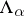is defined to be the limes superior of the quotientas, where. The lower right-hand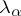, the upper left-hand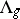, and the lower left-hand Dini derivative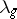are defined analogously. If(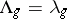), thenhas at the pointa one-sided right-hand (left-hand) Dini derivative. The ordinary derivative exists if all four Dini derivatives coincide. Dini derivatives were introduced by U. Dini . As N.N. Luzin showed, if all four Dini derivatives are finite on a set, then the function has an ordinary derivative almost-everywhere on that set.